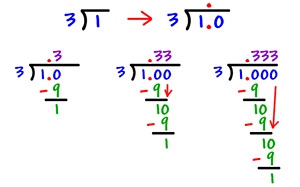# Equivalent Fractions and DecimalsIn this lesson, we will maintain and polish our skills in working with fractions and decimals, by focusing on converting rational numbers to decimals using long division. This conversion will demonstrate the fact that a rational number either terminates or repeats when changed to decimal form. Once we get the hang of converting fractions to their equivalent decimal form, we will jump into the problem-solving involving the four operations with decimals.

Fractions, decimals, and percents are simply different ways of representing the same number. When we think of money, we know that fifty cents is written as \$.50. We also know it as ½ dollar. If anyone asked you what percent of a dollar that amount was, could you tell them?

### Example OneFirst, let us convert a fraction to a decimal number. To convert a fraction to a decimal, divide the numerator by the denominator. One common phrase for remembering this is “box top” because you put the top number in the division box.   Look at the following example to see how to use division to convert a fraction to a decimal.

### Example TwoNow, we can use the same strategy to convert 1/3 to a decimal.

The decimal 0.25 in the example at the top of the page is a terminating decimal because the decimals end. In examples 1 and 2, we can see that the decimal 0.333… repeats its pattern on and on, so it is known as a repeating decimal because the decimal repeats a pattern forever. You can also write a repeating decimal with a bar over the repeating part.

## Practice

Convert the following fractions to decimals.

1)  1/8
2)  1/9
3)  2/3
4)  4/7
5)  3/10
6)  5/3
7)  9/5
8)  5/6

(source)